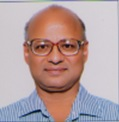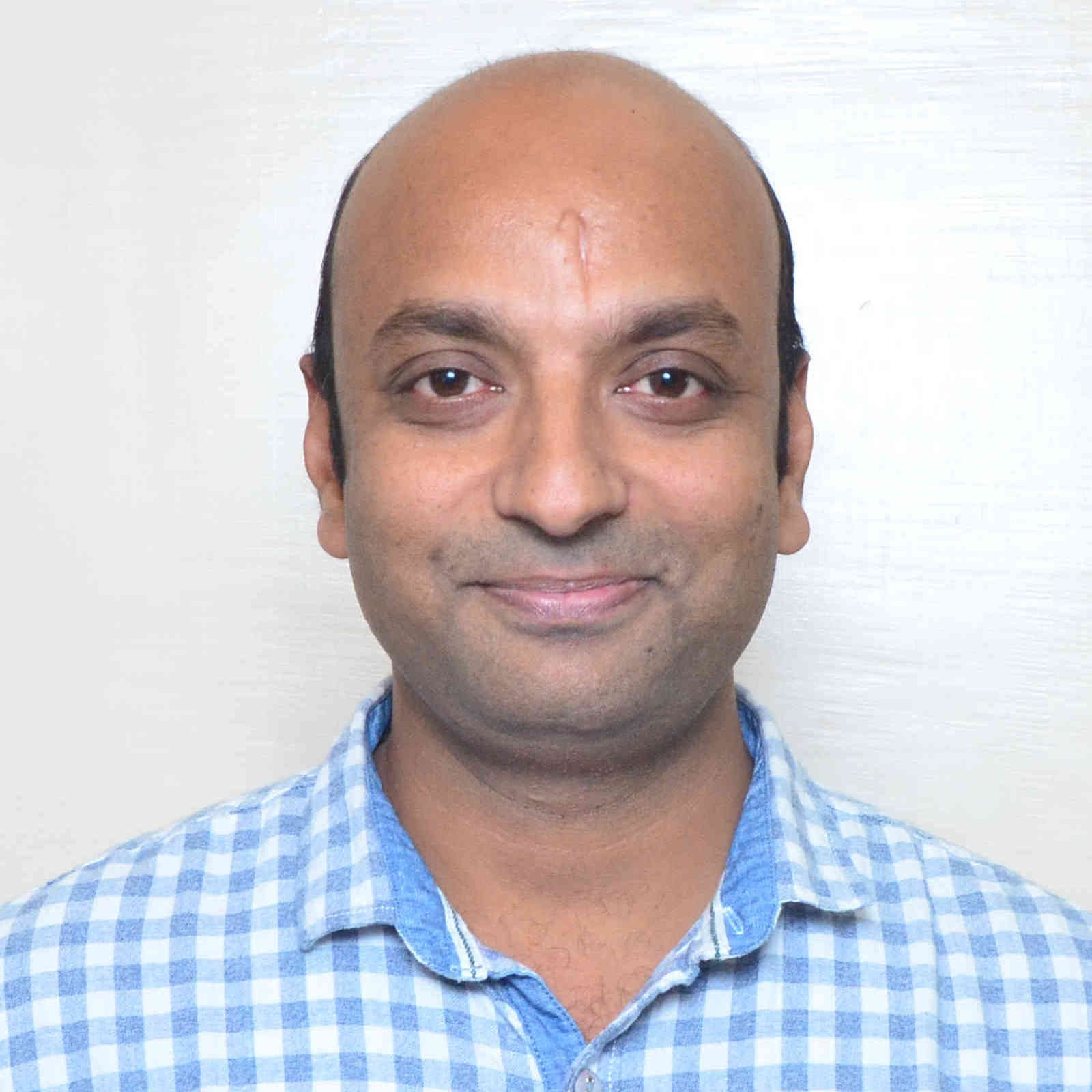# Mathematical Methods and its Applications

By Prof. P. N. Agarwal, Prof. S. K. Gupta   |   IIT Roorkee
Learners enrolled: 834
This course is a basic course offered to UG student of Engineering/Science background. It contains ODE,PDE, Laplace transforms, Z-transforms, Fourier series and Fourier transforms. It plays an important role for solving various engineering sciences problems.Therefore, it has tremendous applications in diverse fields in engineering sciences.

INTENDED AUDIENCE  : UG students of technical universities/colleges.
PRE-REQUISITES  : Nil
INDUSTRY SUPPORT  : Nil
Summary
 Course Status : Ongoing Course Type : Elective Duration : 12 weeks Start Date : 26 Jul 2021 End Date : 15 Oct 2021 Exam Date : 23 Oct 2021 IST Enrollment Ends : 09 Aug 2021 Category : Mathematics Credit Points : 3 Level : Undergraduate/Postgraduate

### Course layout

Week 1: Introduction to linear differential equations ,Linear dependence, independence and Wronskian of functions,Solution of second-order homogeneous linear differential equations with constant coefficients-I,Solution of second-order homogeneous linear differential equations with constant coefficients-II,Method of undetermined coefficients
Week 2: Methods for finding Particular Integral for second-order linear differential equations with constant coefficients-I,Methods for finding Particular Integral for second-order linear differential equations with constant coefficients-II, Methods for finding Particular Integral for second-order linear differential equations with constant coefficients-III,Euler-Cauchy equations,Method of reduction for second-order, linear differential equations
Week 3: Method of variation of parameters , Solution of second order differential equations by changing dependent variable, Solution of second order differential equations by changing independent variable,Solution of higher-order homogenous linear differential equations with constant coefficients, Methods for finding Particular Integral for higher-order linear differential equations
Week 4: Formulation of Partial differential equations, Solution of Lagrange’s equation-I, Solution of Lagrange’s equation-II,Solution of first order nonlinear equations-I,Solution of first order nonlinear equations--II
Week 5: Solution of first order nonlinear equations-III,Solution of first order nonlinear equations-IV,Introduction to Laplace transforms,Laplace transforms of some standard functions,Existence theorem for Laplace transforms
Week 6: Properties of Laplace transforms--I, Properties of Laplace transforms--II, Properties of Laplace transforms--III, Properties of Laplace transforms--IV, Convolution theorem for Laplace transforms--I
Week 7: Convolution theorem for Laplace transforms--II,Initial and final value theorems for Laplace transforms, Laplace transforms of periodic functions, Laplace transforms of Heaviside unit step function,Laplace transforms of Dirac delta function
Week 8: Applications of Laplace transforms-I, Applications of Laplace transforms-II, Applications of Laplace transforms-III,   Z – transform and inverse Z-transform of elementary functions, Properties of Z-transforms-I
Week 9:  Properties of Z-transforms-II, Initial and final value theorem for Z-transforms, Convolution theorem for Z- transforms, Applications of  Z- transforms--I,Applications of Z- transforms-II
Week 10: Applications of Z- transforms--III, Fourier series and its convergence--I, Fourier series and its convergence--II, Fourier series of even and odd functions,Fourier half-range series
Week 11: Parsevel’s Identity, Complex form of Fourier series, Fourier integrals, Fourier sine and cosine integrals, Fourier transforms
Week 12: Fourier sine and cosine transforms,Convolution theorem for Fourier transforms,Applications of Fourier transforms to BVP-I,Applications of Fourier transforms to BVP-II,Applications of Fourier transforms to BVP-III

Nil

### Instructor bio### Prof. P. N. Agarwal

IIT Roorkee
Dr. P. N. Agarwal is a Professor in the Department of Mathematics, IIT Roorkee. His area of research includes approximation Theory and Complex Analysis. He delivered 13 video lectures on Engineering Mathematics in NPTEL Phase I and recently completed Pedagogy project on Engineering Mathematics jointly with Dr. Uaday Singh in the same Department. Further he has completed online certification course “Mathematical methods and its applications” jointly with Dr. S.K. Gupta of the same department. He taught the course on “Integral equations and calculus of variations” several times to MSc (Industrial Mathematics and Informatics) students. He has supervised nine Ph.D. theses and has published more than 187 research papers in reputed international journals of the world. Currently, he is supervising eight research students.### Prof. S. K. Gupta

Dr. S. K. Gupta is an Associate Professor in the Department of Mathematics, IIT Roorkee. His area of expertise includes nonlinear, non-convex and Fuzzy optimization. He has guided three PhD thesis and have published more than 40 papers in various international journals of repute.

### Course certificate

The course is free to enroll and learn from. But if you want a certificate, you have to register and write the proctored exam conducted by us in person at any of the designated exam centres.
The exam is optional for a fee of Rs 1000/- (Rupees one thousand only).
Date and Time of Exams: 23 October 2021 Morning session 9am to 12 noon; Afternoon Session 2pm to 5pm.
Registration url: Announcements will be made when the registration form is open for registrations.
The online registration form has to be filled and the certification exam fee needs to be paid. More details will be made available when the exam registration form is published. If there are any changes, it will be mentioned then.
Please check the form for more details on the cities where the exams will be held, the conditions you agree to when you fill the form etc.

CRITERIA TO GET A CERTIFICATE

Average assignment score = 25% of average of best 8 assignments out of the total 12 assignments given in the course.
Exam score = 75% of the proctored certification exam score out of 100

Final score = Average assignment score + Exam score

YOU WILL BE ELIGIBLE FOR A CERTIFICATE ONLY IF AVERAGE ASSIGNMENT SCORE >=10/25 AND EXAM SCORE >= 30/75. If one of the 2 criteria is not met, you will not get the certificate even if the Final score >= 40/100.

Certificate will have your name, photograph and the score in the final exam with the breakup.It will have the logos of NPTEL and IIT Roorkee.It will be e-verifiable at nptel.ac.in/noc.

Only the e-certificate will be made available. Hard copies will not be dispatched.

Once again, thanks for your interest in our online courses and certification. Happy learning.

- NPTEL team